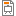# 17ABBITP - Integral Calculus

Code Completion Credits Range Language
17ABBITP Z,ZK 5 2P+2C English
Linear Algebra and Differential Calculus (17ABBLAD)
Lecturer:
Eva Feuerstein (guarantor)
Tutor:
Eva Feuerstein (guarantor)
Supervisor:
Department of Natural Sciences
Synopsis:

The subject is an introduction to integral calculus and integral transforms.

Integral calculus: anti-derivative, indefinite integral, properties and methods of integration (integration by parts and by substitution, partial fractions), definite integral, properties, Newton-Leibnitz fundamental theorem, simple applications of both indefinite and definite integrals, improper integral, solving differential equations (ODEs) (1st order ODEs with separable variables, linear 1st order homogenous as well as non-homogenous ODEs, 2nd order linear homogenous and non-homogenous ODEs with constant coefficients),intro to multiple integrals, particularly double integral and applications.

Integral transforms: Laplace transform and inverse Laplace transform and their application for solving nth order linear ODEs with constant coefficients.

Z-transform and inverse Z-transform, their application for solving nth order linear difference equations.

Requirements:

Assessment:

1. Maximum 3 absences during the semester for serious reason.

2. Activities at seminars will be checked by mini-tests (10 minutes,1-2 tasks). There will be 8 mini-tests during the semester, evaluated by 5 points each. Total sum MT ranging from 0 to 40 points.

3. Midterm tests

1st midterm test in the middle of the current semester (see schedule for the current academic year (AY))

2nd midterm test in the end of the current semester (see schedule for the current AY)

Midterm test consists of 4 tasks, each task evaluated by 5 points (maximum 20 points at a midterm test). A student must gain from one midterm test at least 10 points, it means that minimum gained from both midterm tests must be at least 20 points. So, VT ranging from 20 to 40 points.

Student's grading from seminars transferred for the exam:

MT:8 + VT:4 - ranging from 5 to 15 points.

Additional term for missing midterm tests (see schedule for the current AY)

Exam:

Assessment registered by respective teacher in KOS together with the points transferred for exam.

Exam is only written, lasts 90 minutes.

It is forbidden to use a calculator, mobile phone, or any other electronic device during the exam.

The exam consists of

1. 7 tasks, evaluated by 10 points each, in total maximum 70 points

2. 5 tests, evaluated by 2 points each, in total maximum 10 points

3. 5 tests, evaluated by 1 point each, in total 5 points

4. Evaluation at seminar - transferred points maximum 15 points

A: 90-100, B: 80-89, C: 70-79, D: 60-69, E: 50-59, F: less than 50

Syllabus of lectures:

1. Introduction to indefinite integral, basic properties, elementary functions integration, integration by parts, integration by substitution.

2. Rational functions integration, partial fraction technique.

3. Integration of trigonometric functions, combined techniques of integration.

4. Introduction to definite integral, simple geometrical applications (area, volume of rotational bodies, curve length).

5. Improper integral, introduction to differential equations, general solution.

6. Differential equations, initial value problem for ODEs, 1st order ODE with separable variables, linear 1st order ODEs homogenous and non-homogenous, method of variation of constant, homogenous ODEs (substitution z=y/x).

7. nth order linear ODEs with constant coefficients and their solution.

8. Double integral, introduction and elementary methods of its calculating.

9. Jacobian and substitution in double integral, polar coordinates, geometrical applications of double integral.

10. Laplace transform- definition, properties and examples.

11. Inverse Laplace transform, application of Laplace transform for solving IVP for homogenous and non-homogenous nth order linear ODEs with constant coefficients.

12. Z-transform - definition, properties and examples.

13. Inverse Z-transform, Test No. 2

14. Z-transform for solving linear difference equations.

Syllabus of tutorials:

1. Elementary functions integration, integration by parts, integration by substitution.

2. Rational functions integration, partial fraction technique.

3. Integration of trigonometric functions, combined techniques of integration.

4. Definite integral, simple geometrical applications (area, volume of rotational bodies, curve length).

5. Improper integral, simple examples of improper integrals due to the function or due to the infinite interval of integration, introduction to differential equations, general solution.

6. 1st order ODE with separable variables examples, linear 1st order ODEs homogenous and non-homogenous, method of variation of constant, examples.

7. Homogenous ODEs (substitution z=y/x), nth order linear ODEs with constant coefficients and their solution.

8. Double integral, introduction and elementary methods of its calculating.

9. Jacobian and substitution in double integral, polar coordinates, geometrical applications of double integral.

10. Laplace transform properties and examples.

11. Inverse Laplace transform and application of Laplace transform for solving IVP for homogenous and non-homogenous nth order linear ODEs with constant coefficients.

12. Z-transform properties and examples.

13. Inverse Z-transform. Simple examples.

14. Z-transform for solving linear difference equations.

Study Objective:

The goal of the subject is to gain necessary theoretical background in the field of integral calculus and integral transforms and practical skills in solving various examples and problems of fundamental integral calculus, and integral transforms.

Study materials:

Study materials

 Neustupa J.: Mathematics 1, skriptum ČVUT, 2004

 Bubeník F.: Problems to Mathematics for Engineers, skriptum ČVUT, 2007

 Stewart, J. : Calculus, Brooks/Cole, 2012



Note:
The course is a part of the following study plans:

Lectures:
AttachmentSizeIC_1_2019241.07 KBIC_2-3_2019286.84 KBIC_4_2019177.38 KBIC_5_2019979.97 KBIC_6_2019300.75 KBIC_7_2019665.23 KBIC_8_2019532.42 KBIC_9_2019461.6 KBIC_10_2019466.88 KBIC_11-12_20191.08 MBIC_13_20191.31 MBIC_14_2019360.86 KB

Exercises:
AttachmentSizeITP_SEM1_2020155.69 KBITP_SEM2-3_2020500.67 KBITP_SEM4_2020209.01 KBITP_SEM5-6_2020207.18 KBITP_SEM7_201944.36 KBITP_SEM8_2019382.76 KBITP_SEM9-10_2019371.14 KBITP_SEM11-12_2019363.17 KBITP_SEM13-14_2019306.71 KBTable of integrals, Laplace and Z transforms229.12 KB

Others:
AttachmentSize17ABBITP - Schedule ABBITP AY 2019-20 UPDATED290.39 KB17ABBITP Assessment, Exam Grading AY 2019-20 UPDATED234.3 KB17ABBITP - Topics for exam AY 2019-2020 UODATED168.31 KB17ABBITP - Schedule ABBITP AY 2019-20288.26 KB2nd MIDTERM TEST SAMPLE TASKS 2019358.97 KB1st MIDTERM TEST SAMPLE TASKS 2019255.3 KB